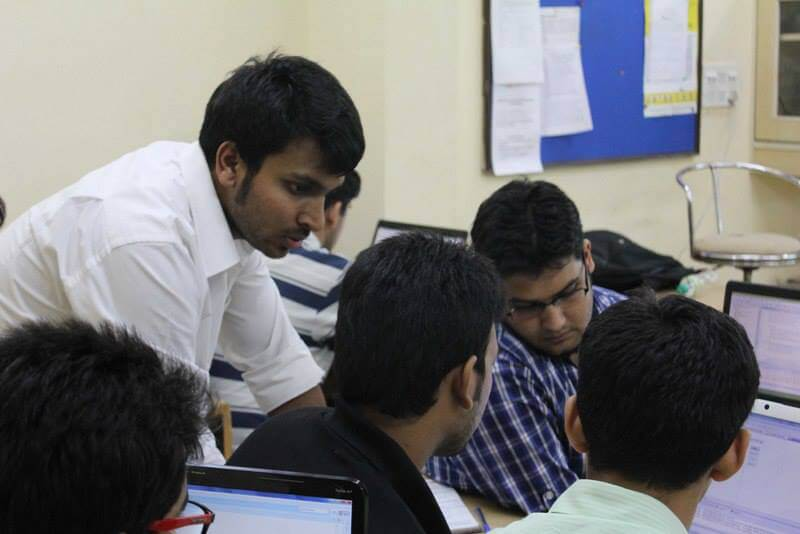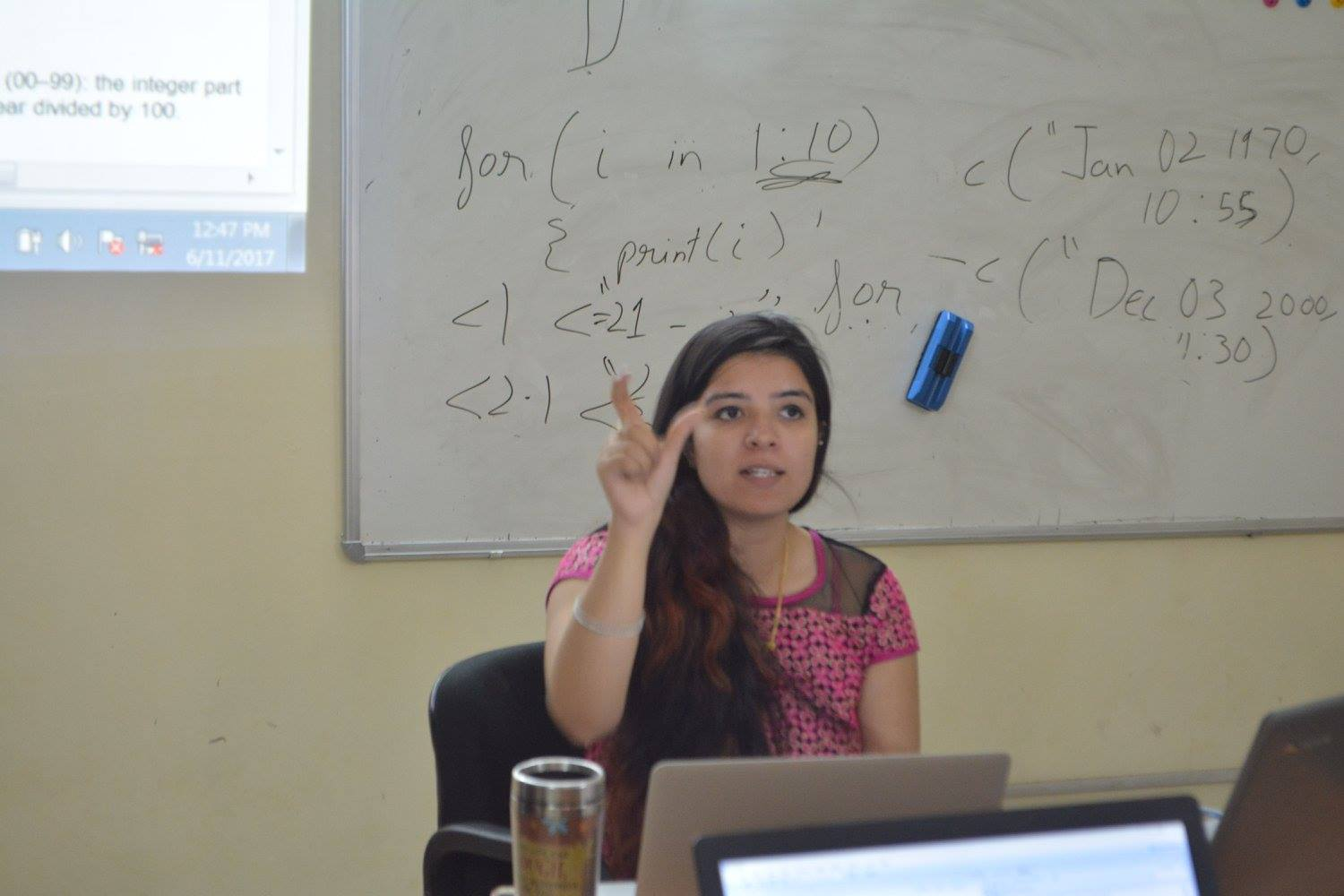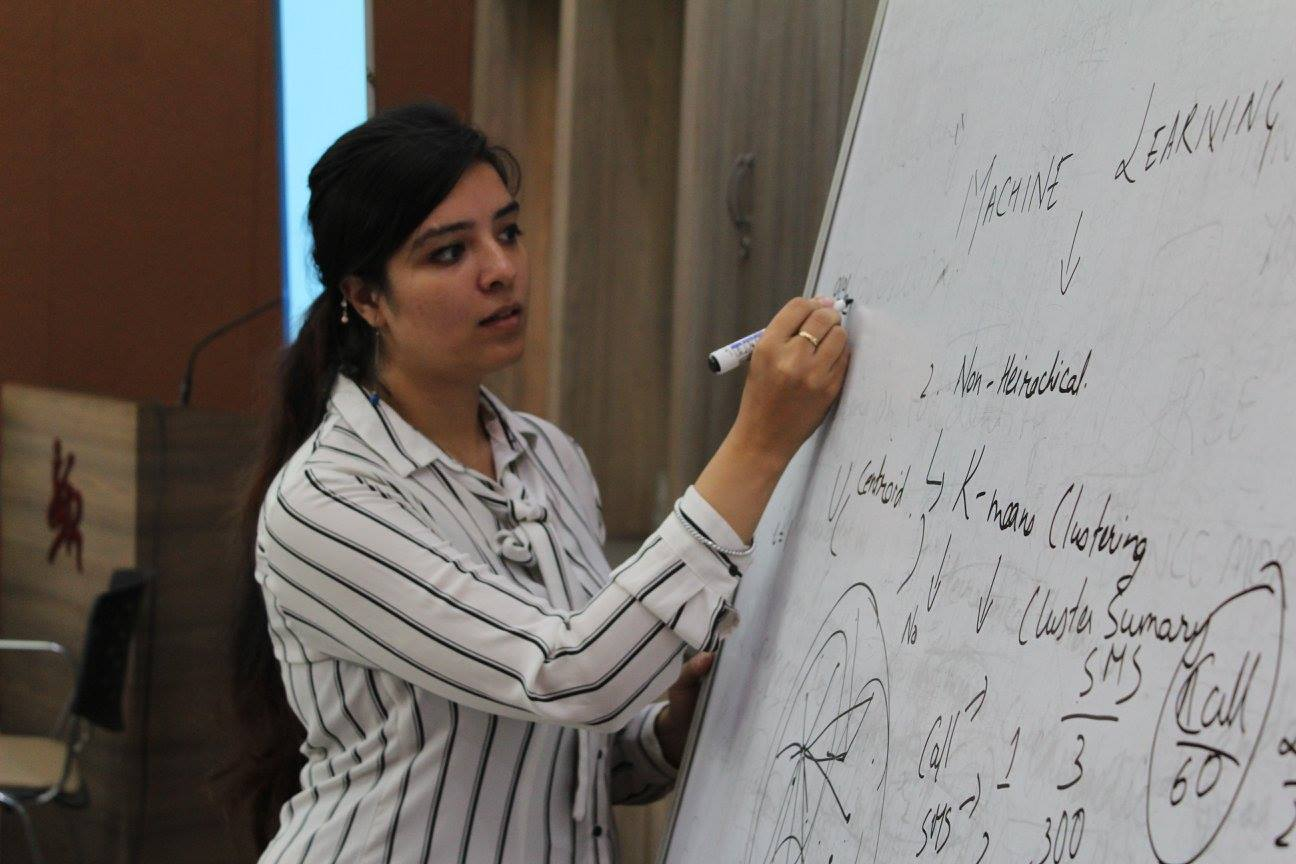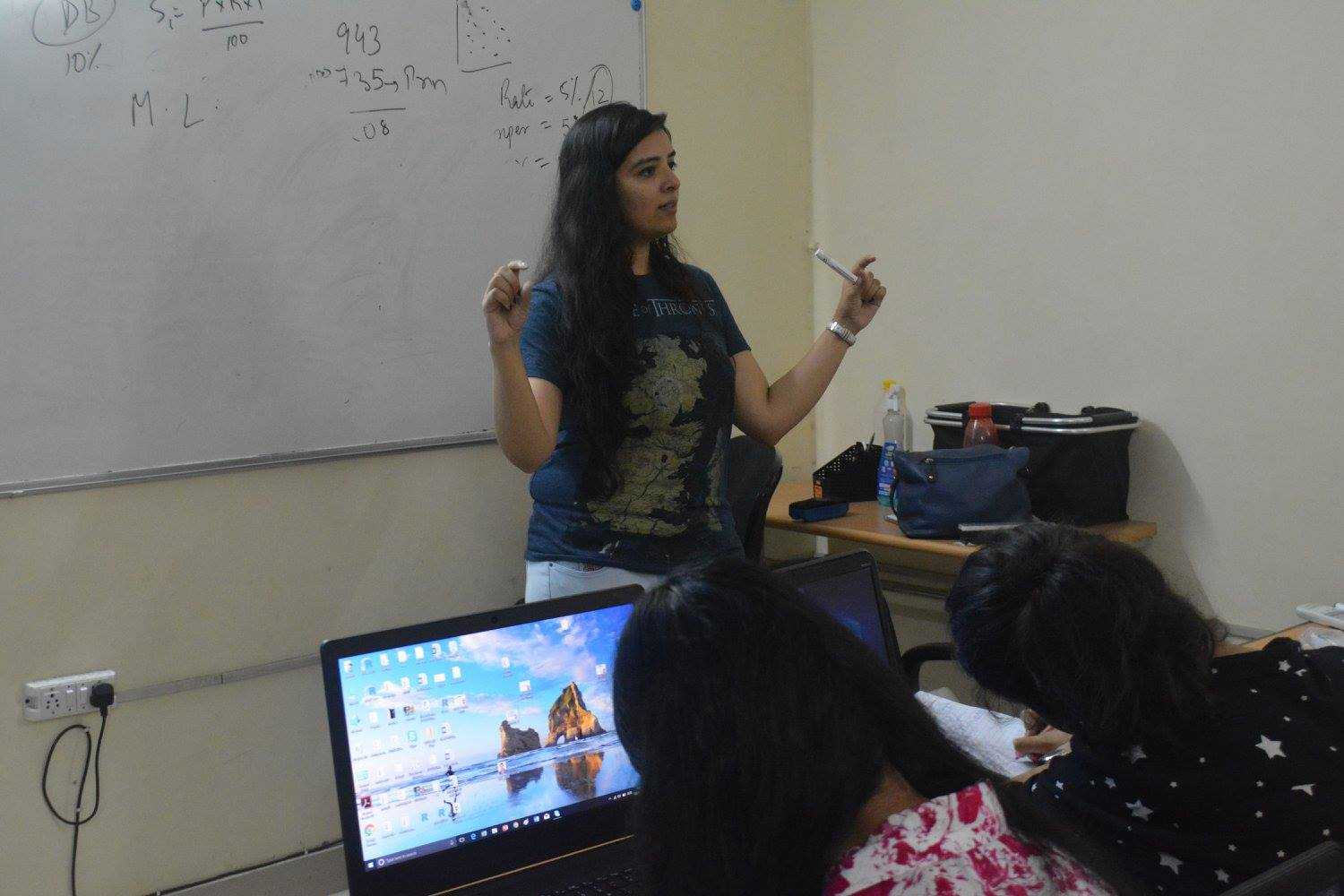## Python

••••#### Python

This course has been designed in two phases. Phase one introduces Python as a programming language and phase two surveys the foundational topics of data science such as data manipulation (using Numpy, Pandas), data communication and visualization (using Matplotlib, Seaborn), and data analysis with Statistics and Machine Learning (using Scikit-Learn).

#### Prerequisites

Basic Knowledge of Statistics will be helpful

#### Faculty Profile

She is a technology and data enthusiast. She is currently working as a Data Scientist at S&P Global Market Intelligence, one of the leading providers of real-time data and analytics to institutional investors and corporations. She has over 3 years of experience in the field of Data Mining and Analytics. She likes to explore interdisciplinary Data Science to utilize technical skills borrowed from computer science and statistics to tackle real-world problems in social media, healthcare, and finance.
Professional Skills: Probability and Statistics, Linear Algebra, Machine Learning, Natural Language Processing, Deep Learning using Python

#### MODULE 1: Introduction to Python

• Introduction to Python
• Why Python? Advances and use in Data Science?
• Getting Started with Anaconda, Spyder
• Writing Basic Programs in Python
• Introduction of Data Types
• How to make functions in Python

#### MODULE 2: Python Programming 1

• Variables, operations, control flow - assignments, conditionals
• Loops, functions
• Python: types, expressions, strings, lists, tuples
• Python memory model: names, mutable and immutable values
• List operations: slices etc
• Text, numeric, date, utility functions in Python

#### MODULE 3: Python Programming 2

• More on Python functions: optional arguments, default values
• Passing functions as arguments, Higher order functions on lists
• Exception handling
• Basic input/output
• Handling files, File I/O, Reading & Writing data to Files, CSV Files
• String processing, String slicing, Testing, searching and manipulating strings

#### MODULE 4: Python Programming 3

• Object Oriented Programming in Python
• Nested functions, Recursive Functions in Python
• Text, numeric, date, utility functions in Python
• Lambda Function in Python
• Introduction & Use of Jupyter Notebooks, Markdown in Jupyter Notebooks

#### MODULE 5: Numpy, Pandas and Matplotlib

• Pandas: Pandas Foundation, Pandas advanced indexing and slicing, Importing Data
• Matplotlib: Exploratory Data Analysis (EDA), Simple & Multiline plots, Customizing plots

#### MODULE 6: Data Analysis and Manipulation

• Advanced Pandas: Groupby, Multi Index, Concat, Merge, Pivot, Pivot table
• Statistical plots with Seaborn: Box plot, Violin plot, Swarm plot, Pair plot, Heatmap etc.
• Data preparation for Machine Learning: Missing values, Categorical data handling, Data transformation, Feature Engineering

#### MODULE 7: Introduction to Machine Learning (ML)

• Introduction to Machine Learning (ML), Types of ML, Introduction to Supervised ML
• Understanding Linear Regression, Implementing Linear Regression in Sci-kit Learn
• Overfitting and Underfitting, Regularized Regression, Logistic Regression

#### MODULE 8: Implementing Advanced Machine Learning Models

• K-Nearest Neighbours, Confusion Matrix, Cross-Validation, Hyper-parameter tuning
• Decision Trees, Ensemble Models: Random Forest, Voter Classifier, AdaBoost

#### MODULE 9: Introduction to Unsupervised Machine Learning

• Introduction to Unsupervised Machine Learning, Implementation of Clustering with KMeans
• Dimensionality Reduction with Principal Component Analysis